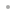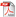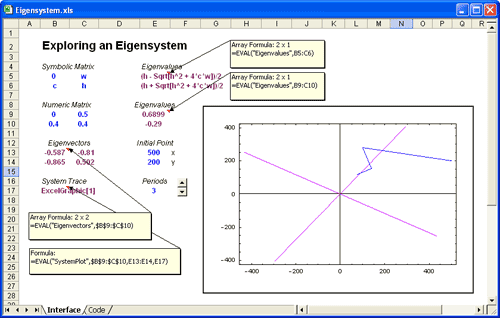MATHEMATICA LINK FOR EXCELMathematica Link for ExcelFeaturesWhat's New in Version 3Who's It For?ExamplesFinanceManagementEngineeringIndustryUser's Manual and Reference GuideBuy Online# Examples: Engineering

Engineering calculations that Excel is unable to perform alone can be done using Mathematica. In this example, we calculate eigenvalues and eigenvectors for a 2x2 matrix. Concise symbolic results are obtained from the matrix, as shown at the top of the worksheet. In the rest of the worksheet, we use numeric calculations to determine system behavior, and a Mathematica graphic plots the system's eigenvectors and projected path.

On its own, Excel has a few hundred worksheet functions. Mathematica Link for Excel extends your reach to thousands of Mathematica functions, covering diverse areas of mathematics, engineering and science.